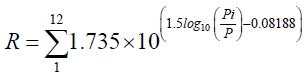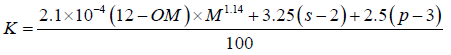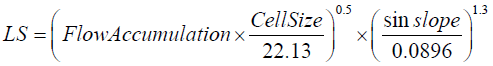All submissions of the EM system will be redirected to Online Manuscript Submission System. Authors are requested to submit articles directly to Online Manuscript Submission System of respective journal.Hazem Ghassan Abdo1,2,3* and Rania Mohssen Hassan4,5

1 Department of Geography, Damascus University, Damascus, Syrian Arab Republic, Email: [email protected]
2 Department of Geography, Tishreen University, Lattakia, Syrian Arab Republic
3 Department of Geography, Tartous University, Tartous, Syrian Arab Republic
4 Department of Statistics and Programming Tishreen University, Lattakia, Syrian Arab Republic
5 Department of Statistics and Programming, Tartous University, Tartous, Syrian Arab Republic

*Correspondence: Hazem Ghassan Abdo, Department of Geography, Damascus University, Damascus, Syrian Arab Republic, Tel: +963936971611, Email: [email protected]

Received Date: Oct 23, 2018 / Accepted Date: Dec 07, 2018 / Published Date: Dec 23, 2018

Citation: Abdo HG, Hassan RM. A Statistical-spatial modeling of soil erosion: case study of Al-Sen basin, Tartous, Syria. J Environ Geol. 2018;2(2):68-74.

This open-access article is distributed under the terms of the Creative Commons Attribution Non-Commercial License (CC BY-NC) (http://creativecommons.org/licenses/by-nc/4.0/), which permits reuse, distribution and reproduction of the article, provided that the original work is properly cited and the reuse is restricted to noncommercial purposes. For commercial reuse, contact [email protected]

## Abstract

Al-Sen watershed with a surface area of 98.8 km2 is forming an important vital spatial sector for Syrian Coast Basin in the western part of Syria. Soil loss in Al-Sen basin is one of the major growing eco-environmental risks that need to be assessed geographically. Statistical-Spatial evaluation of soil erosion risks is a useful and urgent step for creating soil erosion management plans along with spatial implementation. At present study, a quantitative assessment of soil erosion on grid basis is carried out using GIS and SPSS technologies. The outcomes illustrate that 43.01% of the study area affected by low erosion of soil, 29.05% moderate erosion, 16.33% high erosion, and 11.85% very high erosion, respectively. Moreover, the major factor accelerating soil loss in the study basin was LS factor of RUSLE (r2=0.74). This study, however, has also suggested two statistical models of the soil erosion case in an Al-Sen basin. The results of this study are very important for local decision-makers in establishing spatial conservation strategies.

### Keywords

Soil erosion, RUSLE, Statistical-spatial modeling, Al-Sen basin, Syria

Soil is the effective part of the top layer of the Earth’s crust, ranging in thickness from a few centimeters to several decameters which is considered one of the human continuation principle worldwide [1,2]. Soil erosion is a dynamic complex process defined as separating, transferring, and accumulating surface soil products through water and/or wind agents. Soil erosion affected by raindrops or wind energy, physical and chemical properties, terrain, and land cover . Soil erosion causes many negative consequences on land productivity, nutrient depletion, soil quality degradation, and reduced farm income, due to loss of fertility elements by soil mobilization [4-7].

Mainly, the soil erosion by water endangers the ecosystem of wet territories. Syrian Coastal Region (SCR) generally and Al-Sen basin particularly represent a favorable geomorphologic, lithologic, hydro-climatic and socio-economic model for acceleration of soil erosion risks as most researches refer [2,8-10]. Al-Sen basin deems the most important vital, economic, and agricultural pole in SCR. Al-Sen basin is prone to huge amounts of soil erosion. The basic aim of this research is the statistical-spatial modeling of soil loss by utilizing the RUSLE approach through GIS and SPSS Techniques in Al-Sen basin. However, this issue is very important and urgent need to taking the conservation measures with spatial applying swiftly.

### Materials and Methods

Study area

Al-Sen River Basin (ASRB) is located in Tartous province, in the western part of Syria with 98.8 km2 area (Figure 1). ASRB extends astronomically between the latitudes of 35 ̊.3՛ and 35 ̊.22՛ North and longitude of 35 ̊.9՛ and 36 ̊.17՛ east. Geographically, ASRB boarded by Coast Mountains from the east, the Mediterranean from the west, Hrisoon basin from the south, and Al-Berghel basin from the north. Geomorphologically, the basin can be divided into three basic landforms sectors. The mountainous, hill and plain sectors, with altitudes ranging from 0-100 m, 100-400 m, and 400-1257 m, respectively. Soil erosion rates, in this context, in the mountainous and hill regions are increasing due to steep slopes. The study area is mainly subject to the Mediterranean climate. The annual rainfall rate is about 1000 mm. Most of the rain falls during winter from November to May. The mean annually temperature is ranging 13.4 °C to 18.7 °C (Figure 2).

Figure 2) Spatial distribution of rainfall values

Calculation of RUSLE factors

The universal equation of soil loss (RUSLE) is the most widely utilized empirical formula and approach around the world to assess the soil loss per area unit. RUSLE refers to the influence of kinetic raindrops, physical and chemical topsoil properties, terrain features, vegetation cover and conservation criteria in soil loss. RUSLE is given by the following equation :

A = R× K × LS ×C× P (1)

where A is the average annual soil loss in tons per ha per year (t/ha/year), R is the rainfall erosivity, K is the soil erodibility, LS is the hill slope length and steepness, C is the vegetation factor, and P is the support practice.

R factor

The rainfall erosivity (R) factor represents the effect of kinetic raindrops on soil loss . R-value is highly influenced by the body distribution, intensity, speed, amount, time and pattern of rainfall whether for one or more of storms and by the mass of the resulting runoff [13,14]. R factor index within the RUSLE model should be calculated by multiplying the amount raindrops energy (E) and the maximum 30 min. rainfall intensity (I30)  in case of finding data. At the current paper, monthly rainfall data of 47 years (1970–2017) were obtained to map the R-factor by the following Equation 2 developed by Wischmeier and Smith :(2)

Where R is a rainfall erositivity factor (MJ mm ha-1 h-1 per year); Pi is monthly rainfall (mm); P is an annual rainfall (mm). There are five climatic stations established in and around the Al-Sen basin as shown in Figure 1 and Table 1. However, the annual rainfall and R factor values were mapped with the Inverse Distance Weighted Average (IDW) interpolation method in the GIS as shown in Figures 2 and 3, respectively. In the study area, the spatial R-values were ranging between 4.3 and 42.3 MJ mm ha-1 year-1 .

Climatic station Total annual rain fall (m) R factor (MJ mm ha-1 h-1 year-1)
Banias 809 4.4
Doir-Babdah 977 14.5
Ain-Alsharqia 1007 24.7
Shendakha 1162 31.9
Mnizla 1241 42.1

Table 1: Climatic stations used in the study with R factor values for each station

Figure 3) Spatial distribution of R factor values

K factor

K factor is an important field parameter in assessing the risk of soil erosion. K factor examines the susceptibility of a soil to loss based on the physical and chemical characteristics of collected and soil samples as measured under the standard unit plot condition. The standard condition is the unit plot, 22.6 m long with a 9% gradient, maintained in continuous fallow, tilled up and down the hill slope [16,17]. The results of analyzed soil samples in Beit-kammouneh Center for Agricultural Center in Tartous Governorate utilized for mapping K factor by utilized the following equation conducted by Wischmeier et al. :(3)

Where OM is the organic matter (%), s is soil structure class, p is permeability class, and M is aggregated variable derived from the granular soil texture: M=(%Msilt) × (%silt+%sand), and the modified silt (Msilt) is a percentage of grain size between 0.002 and 0.1 mm. By utilizing a previous equation, the spatial K-values (tons MJ-1 hmm-1) were computed and subsequently, the K factor map was generated (Figure 4).

Figure 4) Spatial distribution of K factor values

LS factor

LS factor has explored the influence of topographical features on the spatial acceleration of soil loss. Slope length (L) is determined as the horizontal distance from the origin of overland flow to the point where either the slope gradient decreases enough that deposition begins or runoff becomes concentrated in a defined channel. The slope steepness (S) factors represent the impact of slope gradient on soil loss . However, the effects of slope steepness have a high influence on soil erosion than slope length. By utilizing a Digital Elevation Model (DEM) with 30 m resolution  LS factor in the study basin was mapped depending on the following Equation 4:(4)

Where Flow Accumulation is the grid layer of flow accumulation expressed as the number of grid cells, and Cell Size is the length of a cell side. Spatial LS-values of the study basin are ranging between 0- 22.19 (Figure 5).

Figure 5) Spatial distribution of LS factor values

C factor

Vegetation has a considerable effect on the runoff and soil loss rates by alleviating the kinetic energy of raindrops side-by-side with decreasing runoff speed. C-factor is defined as the proportion of soil loss from the plot with appointed vegetation to the conformable soil erosion from ongoing flux [12,20]. There are two methods to make C-factor, the first one depending on land use/land cover, and the other depending on the normalized difference vegetation index (NDVI) [9,21]. C factor raster map, hence in the current study, was generated based on NDVI index. NDVI index is defined as the ratio of the difference between the Near-Infrared Band (NIR) and the red band (R) and the sum of these two bands . NDVI was calculated depending on Landsat 8 that taken at May 2018 through the following equation:

NDVI = (NIR - RED) / (NIR + RED) (5)

Where NIR is the near-infrared band (band 4, 0.76–0.90 μm), and RED is the red band (band 3, 0.63–0.69 μm). NDVI values range between-0.11 and 0.54 as shown in Figure 6. Continuously, C factor raster map as shown in Figure 7 can be generated by the following equation [23,24]:

Figure 6) Spatial distribution of NDVI values in May 2018

Figure 7) Spatial distribution of C factor values based on NDVI indicator

P factor

P factor, or the maintenance practice, is the proportion of soil loss after a specific conservation measure to the dredged soil after up and down plowing . These practices basically mitigate soil loss by adjusting the flow system, flux intensity and kinetic or trend of runoff. Moreover, the kind of practice, such as terracing, contour plowing, and regular barriers, mitigate the rate of soil loss effectively. The value of the P-factor is ranging 0-1, the value reaching to 0 indicates well conservation measures, and the value reaching to 1 indicates poor conservation measures . Field inspections indicate the absence of active conservation procedures of lands in the study basin. In order to neglect the P factor from soil erosion estimation, P equals to 1 as suggested by .

### Results and Discussion

In a GIS environment, the soil loss map was produced by spatial multiplication process of the raster maps of RUSLE factors (R, K, LS, and C). In this context, soil erosion values in Al-Sen basin range from 0-18 ton/ha/ yr. Figure 8, however, represents the spatial pattern of soil loss hazards grades. Spatially, the spatial propagation of high grades of loss is distributed in the central and eastern basin, i.e. in the hills and mountainous region, whilst low grads of erosion were distributed in the western lowland. Quantitatively, Table 2 shows the areas of soil erosion risks. The outcomes indicate that about 11% was estimated to have very high loss rates, 16.33% was predicted to have high loss rates, 29.05% has moderate loss rates, and 43% has low loss rates, respectively. The estimated amount of soil loss in Al-Sen basin can be deemed critical according to the fact that the amount of soil erosion tolerant that will allow sustaining an economic, along with a high level of agricultural productivity is ranging from 5 to 11 Mg ha-1 year-1 in Mediterranean regions [26-28]. In this context, conservation criteria must be adopted to control soil loss in areas of high to very high erosion swiftly.

Soil erosion class Rat of soil erosion class in ton/ha/year Area (Km2) Percentage (%)
Low >5 42.5 43.01
Moderate 5-10 28.71 29.05
High 10-15 16.14 16.33
Very high 15-18.12 11.45 11.58

Table 2: Classification of soil erosion in study basin

Figure 8) Soil erosion map in Al-sen basin based on RUSLE model

In SPSS environment, the statistical process was done to determine the most affected RUSLE factor which enhances the acceleration of soil erosion in Al-Sen basin. Statistical correlations and regression relationships between RUSLE parameters and the soil erosion rate for the study basin are introduced in Table 3. It can be considered that the strongest correlation is between the LS factor and annual soil erosion value (r2=0.74) whilst the correlations between other factors of RUSLE and annual soil erosion are found to be very low. In this context, the slope degree of the basin has reached more than 55% as shown in Figure 9. Moreover, Table 4 illustrates the suggested statistical models. In details, the Enter model has excluded K factor, while the Stepwise model has excluded K, R, and C factors, respectively.

RUSLE factors Annual soil erosion
R r2=0.23 y=3.96x-153.4
K r2=0.23 y=102.8x+15
LS r2=0.23 y=2.66x+0.44
C r2=0.23 y= 46.3x-34.6

Table 3: The correlation and regressions among the annual soil erosion and RUSLE factors for the study area

Statistical modelling approach  Statistical model
Enter y=2.076 LS+0.272 R+21.872 C-128.669
Stepwise y=2.034 LS-4.037

Table 4: The statistical models for the study area

Figure 9) Spatial distribution of slope degrees values

### Conclusion

The current study sought to statistical-spatial modeling of soil loss in Al-Sen basin by utilizing the RUSLE approach in GIS and SPSS techniques. The acceleration of soil loss hazards in the study area was clear, which reached in some areas to more than 18t/ha/year. A statistical investigation was conducted also to specify the most essential influential factor in soil loss. Thus, the output visual interpretation is an urgent necessity for immediate implementation in soil maintenance planning and spatial application.

### Acknowledgement

The authors gratefully thank rector of Tartous University Prof. Dr. Issam Al-dali for unlimited academic support. Sincerely thanks also go Prof. Dr Mohammad Akrosh, Prof. Dr. Fatina Yaseen Alshaal and Prof. Dr. Juliet Salloum for academic support. The author express their deep and sincere thanks to the anonymous reviewer and the editor for their valuable comments, suggestions, assistance and constructive criticism in the improvement of earlier version of the manuscript.

### Conflict of Interest

On behalf of all authors, the corresponding author states that there is no conflict of interest.

## Recommended Conferences

London, UK

### World Congress on Earth Science and Environmental Science

San Francisco, USA

### 2nd International Conference on Oil and Gas

Dubai, UAETop